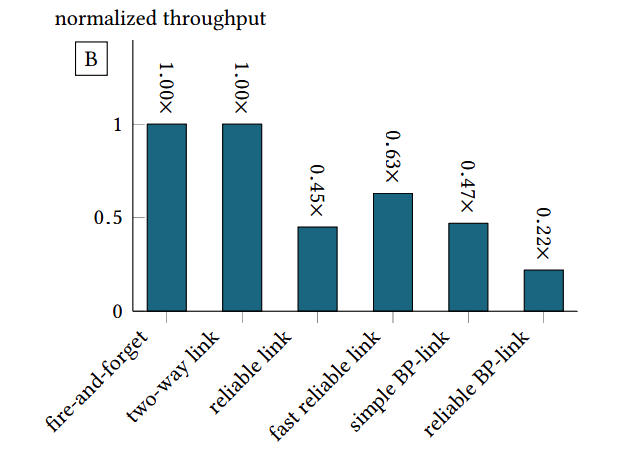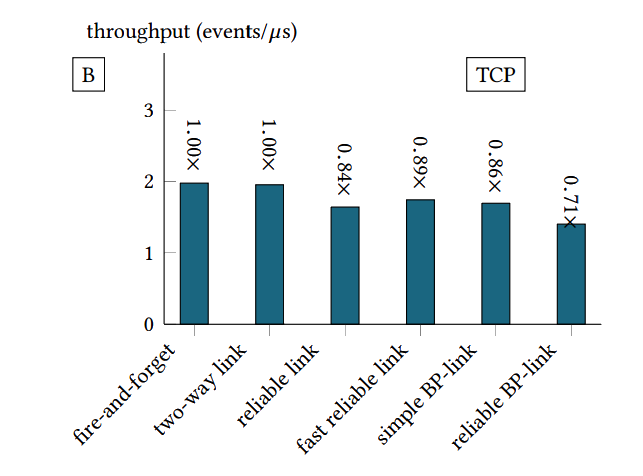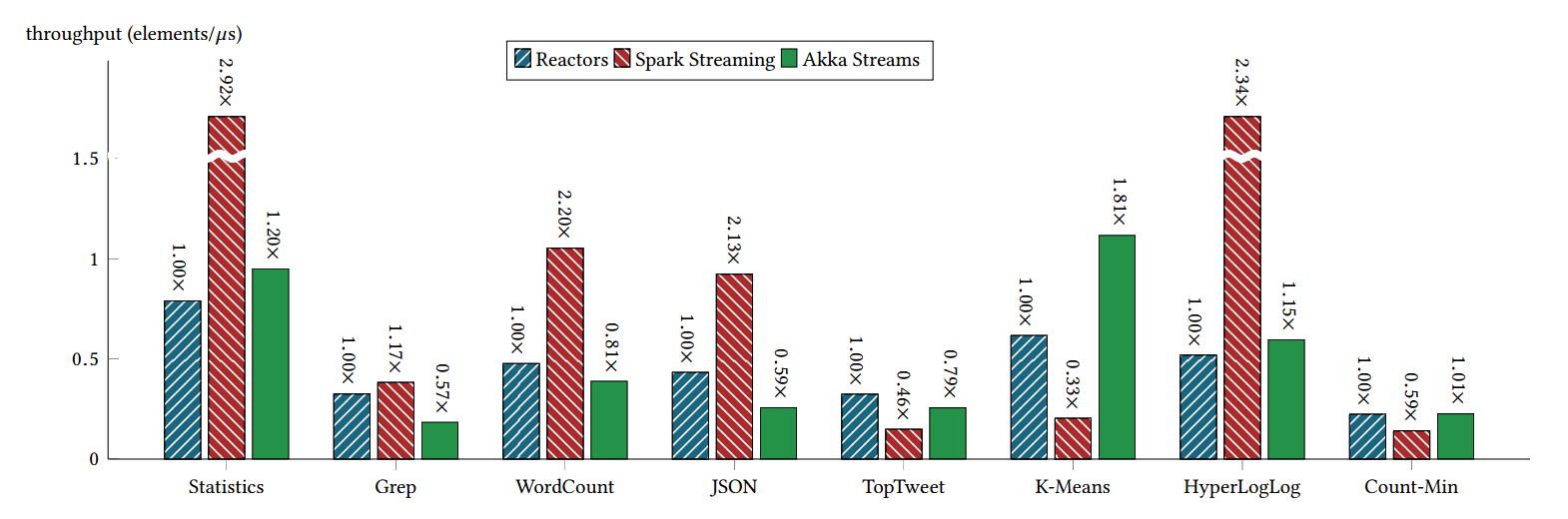Your browser doesn't support the features required by impress.js, so you are presented with a simplified version of this presentation.

For the best experience please use the latest Chrome, Safari or Firefox browser.

## Encoding the Building Blocks of Communication

Oracle Labs Switzerland

October 2017## Safe Harbour

The following is intended to provide some insight into a line of research in Oracle Labs. It is intended for information purposes only, and may not be incorporated into any contract.

It is not a commitment to deliver any material, code, or functionality, and should not be relied in making purchasing decisions. Oracle reserves the right to alter its development plans and practices at any time, and the development, release, and timing of any features or functionality described in connection with any Oracle product or service remains at the sole discretion of Oracle.

Any views expressed in this presentation are my own and do not necessarily reflect the views of Oracle.Should a message-based programming model have backpressure as a core primitive?

## Reactor model

Syntax

```            ```
terms ::=
...
spawn(t)
t ! t
t.onEvent(t)
open[T]
```
```

```            ```
types ::=
...
Events[T]
Channel[T]

```
```## Reactor model

Semantics

```            ```
P = { R }

```
```

```            ```
program

```
```

## Reactor model

Semantics

```            ```
P = { R }

```
```## Reactor model

Semantics

```            ```
P = { R }
R = (t, { C })

```
```

```            ```
program
reactor

```
```

## Reactor model

Semantics

```            ```
P = { R }
R = (t, { C })

```
```## Reactor model

Semantics

```            ```
P = { R }
R = (t, { C })
C = (c, e, x*, F)

```
```

```            ```
program
reactor
connector

```
```

## Reactor model

Semantics

```            ```
P = { R }
R = (t, { C })
C = (c, e, x*, F)

```
```## Reactor model

Semantics

```            ```
P = { R }
R = (t, { C })
C = (c, e, x*, F)
F = { f }
```
```

```            ```
program
reactor
connector
callbacks
```
```

## Reactor model

Semantics

```            ```
P = { R }
R = (t, { C })
C = (c, e, x*, F)
F = { f }
```
```## Question

How to encode a function that returns a backpressure channel?

```        ```
def backpressure[T]: BackpressureChannel[T]
```
```

## Idea

Encode backpressure as a composition of several simpler protocols.

## Client-Server Protocol```        ```
type Req[T, S] = (T, Channel[S])

```
```
```        ```
type Req[T, S] = (T, Channel[S])

type Server[T, S] = Channel[Req[T, S]]

```
```
```        ```
type Req[T, S] = (T, Channel[S])

type Server[T, S] = Channel[Req[T, S]]

def server(f: T => S): Server[T, S] = {

}
```
```
```        ```
type Req[T, S] = (T, Channel[S])

type Server[T, S] = Channel[Req[T, S]]

def server(f: T => S): Server[T, S] = {
val c = open[Req[T, S]]

}
```
```
```        ```
type Req[T, S] = (T, Channel[S])

type Server[T, S] = Channel[Req[T, S]]

def server(f: T => S): Server[T, S] = {
val c = open[Req[T, S]]
c.events onEvent {
case (x, ch) => ch ! f(x)
}

}
```
```
```        ```
type Req[T, S] = (T, Channel[S])

type Server[T, S] = Channel[Req[T, S]]

def server(f: T => S): Server[T, S] = {
val c = open[Req[T, S]]
c.events onEvent {
case (x, ch) => ch ! f(x)
}
c.channel
}
```
```
```        ```
def ?[T, S]
(s: Server[T, S], x: T): Events[S] = {

}

```
```
```        ```
def ?[T, S]
(s: Server[T, S], x: T): Events[S] = {

}

```
```
```        ```
def ?[T, S]
(s: Server[T, S], x: T): Events[S] = {

}

```
```
```        ```
def ?[T, S]
(s: Server[T, S], x: T): Events[S] = {
}

```
```
```        ```
def ?[T, S]
(s: Server[T, S], x: T): Events[S] = {
}

val data = mutable.Map(1 -> 2, 3 -> 4)
val s = server(data)

```
```
```        ```
def ?[T, S]
(s: Server[T, S], x: T): Events[S] = {
}

val data = mutable.Map(1 -> 2, 3 -> 4)
val s = server(data)

(s ? 1) onEvent { x =>
println(x)
}
```
```

## Two-way channels```        ```
type TwoWay[I, O] = (Channel[O], Events[I])
```
``````        ```
type TwoWay.Req[I, O] =
Req[Channel[I], Channel[O]]

```
```
```        ```
type TwoWay.Req[I, O] =
Req[Channel[I], Channel[O]]

type TwoWay.Server[I, O] =
Server[Channel[I], Channel[O]]
```
``````        ```
type TwoWay[I, O] = (Channel[O], Events[I])

```
```
```        ```
type TwoWay[I, O] = (Channel[I], Events[O])

def twoWayServer[I, O]:
(TwoWay.Server[I, O], Events[TwoWay[O, I]])

```
```
```        ```
type TwoWay[I, O] = (Channel[I], Events[O])

def twoWayServer[I, O]:
(TwoWay.Server[I, O], Events[TwoWay[O, I]]) = {
val c = open[TwoWay.Req[I, O]]

}
```
```
```        ```
type TwoWay[I, O] = (Channel[I], Events[O])

def twoWayServer[I, O]:
(TwoWay.Server[I, O], Events[TwoWay[O, I]]) = {
val c = open[TwoWay.Req[I, O]]
val connections = c.events map {
case (input, sender) =>

}

}
```
```
```        ```
type TwoWay[I, O] = (Channel[I], Events[O])

def twoWayServer[I, O]:
(TwoWay.Server[I, O], Events[TwoWay[O, I]]) = {
val c = open[TwoWay.Req[I, O]]
val connections = c.events map {
case (input, sender) =>
val output = open[O]
sender ! output.channel
(input, output.events): TwoWay[O, I]
}

}
```
```
```        ```
type TwoWay[I, O] = (Channel[I], Events[O])

def twoWayServer[I, O]:
(TwoWay.Server[I, O], Events[TwoWay[O, I]]) = {
val c = open[TwoWay.Req[I, O]]
val connections = c.events map {
case (input, sender) =>
val output = open[O]
sender ! output.channel
(input, output.events)
}
(c.channel, connections)
}
```
``````        ```
def connect[I, O](
s: TwoWay.Server[I, O]
): Events[TwoWay[I, O]] = {

}
```
```
```        ```
def connect[I, O](
s: TwoWay.Server[I, O]
): Events[TwoWay[I, O]] = {
val c = open[I]

}
```
```
```        ```
def connect[I, O](
s: TwoWay.Server[I, O]
): Events[TwoWay[I, O]] = {
val c = open[I]
(s ? c) map { output =>
(output, c.events)
}
}
```
``````        ```
type ReliableChannel[T] =
TwoWay[Stamp, (T, Stamp)]

```
```
```        ```
type ReliableChannel[T] =
TwoWay[Stamp, (T, Stamp)]

type ReliableEvents[T] =
TwoWay[(T, Stamp), Stamp]
```
``````        ```
def reliable(e: ReliableEvents[T]): Events[T]
```
``````        ```
def reliable(c: ReliableChannel[T]): Channel[T]
```
``````        ```
type BackpressureEvents[T] =
(Events[Boolean], Queue[T])

```
```
```        ```
type BackpressureEvents[T] =
(Events[Boolean], Queue[T])

def backpressure[T]
(t: TwoWay[T, Int]): BackpressureEvents[T]
```
``````        ```
type BackpressureChannel[T] =
(Channel[T], Events[Boolean])

```
```
```        ```
type BackpressureChannel[T] =
(Channel[T], Events[Boolean])

def backpressure[T](t: TwoWay[Int, T]):
BackpressureChannel[T]
```
``````        ```

Channel[TwoWay.Server[Int, T]]
```
```
```        ```
type Stream[T] =
Channel[TwoWay.Server[Int, T]]
```
```
```        ```
def map[T, S](
s: Stream[T], f: T => S): Stream[S]

def filter[T](
s: Stream[T], f: T => Boolean): Stream[T]

def scanLeft[T,S](
s: Stream[T], z: S,
op: (S, T) => S): Stream[S]

def batch(s: Stream[T], x: Int): Stream[Seq[T]]
```
```
```        ```
def lift[T, S]
(f: Events[T] => Events[S]):
Stream[T] => Stream[S]
```
```## Conclusion

1. Backpressure not a core primitive.

2. However, compiler optimizations might be necessary.

## Thank you!

Use a spacebar or arrow keys to navigate